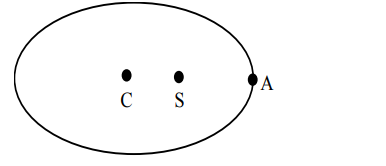# Solve this following`
Question:

Let $\mathrm{E}$ be an ellipse whose axes are parallel to the co-ordinates axes, having its center at $(3,-4)$, one focus at $(4,-4)$ and one vertex at $(5,-4)$. If $m x-y=4, m>0$ is a tangent to the ellipse $E$, then the value of $5 \mathrm{~m}^{2}$ is equal to

Solution:

Given $C(3,-4), S(4,-4)$and $\mathrm{A}(5,-4)$

Hence, $a=2 \& a e=1$

$\Rightarrow \mathrm{e}=\frac{1}{2}$

$\Rightarrow \mathrm{b}^{2}=3$

So, $\mathrm{E}: \frac{(\mathrm{x}-3)^{2}}{4}+\frac{(\mathrm{y}+4)^{2}}{3}=1$

Intersecting with given tangent.

$\frac{x^{2}-6 x+9}{4}+\frac{m^{2} x^{2}}{3}=1$

Now, $D=0$ (as it is tangent)

So, $5 \mathrm{~m}^{2}=3$